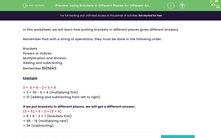# Using Brackets in Different Places for Different Answers

In this worksheet, students practise how to use brackets in an expression.Key stage:  KS 3

Curriculum topic:   Number

Curriculum subtopic:   Use Conventional Notation for Priority of Operations

Difficulty level:#### Worksheet Overview

In this worksheet, we will learn how putting brackets in different places gives different answers.

Remember that with a string of operations, they must be done in the following order:

Brackets
Powers or indices
Multiplication and division

Remember BIDMAS

Example

3 +  5 × 6 - 2 × 3 + 4

= 3 + 30 - 6 + 4 (multiplying first)
= 31 (adding and subtracting from left to right)

If we put brackets in different places, we will get a different answer.

(3 + 5) × 6 - 2 × (3 + 4)

= 8 × 6 - 2 × 7 (brackets first)
= 48 - 14 (multiplying next)
= 34 (subtracting)

### What is EdPlace?

We're your National Curriculum aligned online education content provider helping each child succeed in English, maths and science from year 1 to GCSE. With an EdPlace account you’ll be able to track and measure progress, helping each child achieve their best. We build confidence and attainment by personalising each child’s learning at a level that suits them.

Get started